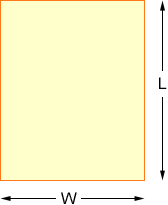Name: Rachel Who is asking: Student Level: Middle Question: a rectangular picture frame has a perimiter of 44.2 cm.The width of the frame is seven tenths of its length what are the dimensions of the frame? Hi Rachel, Draw a diagram. Label the length as L cm and the width as W cm.The perimeter is the total distance around the frame. There are two sides of length L cm and two sides of length W cm. Hence the perimeter is 2L + 2W. That is 2L + 2W = 44.2 ou also know that "The width of the frame is seven tenths of its length", hence W = 7/10 L. Thus 2L + 2( 7/10 L) = 44.2 Solve this equation for L and then use the fact that W = 7/10 L to find W. Cheers, Penny Go to Math Central